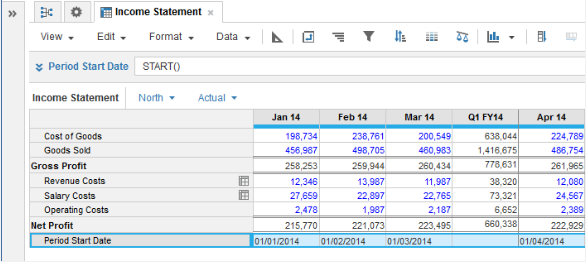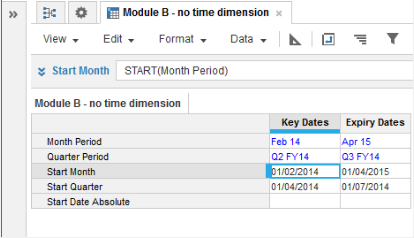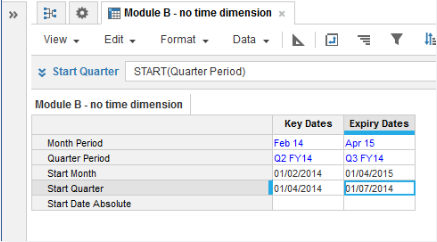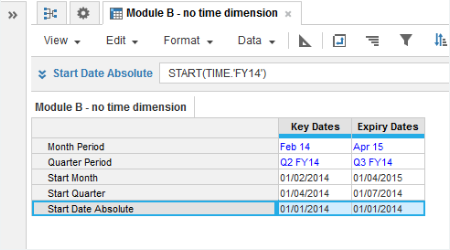1. Calculation functions
2. All Functions
3. Time and Date Functions
4. STARTResult is the first date in the period.

The function can take one optional time period parameter:

• No parameter - Returns first date with reference to the source module time dimension.
• Parameter- can be time period or hard-coded.
• Time period - Returns the first date of the source time period.
• Hard-coded - Returns the first date with reference to the source timescale settings.

## Syntax

```START()
```
`START(x)`

where:

• x: time period

## Format

Input Format Output Format

x: Time period

Date

## Arguments

The function uses the following arguments:

• x: Date: Time period-formatted date variable or line item, hard-coded date.

## Constraints

The function has the following constraints:

• If taking a parameter, source must be time period-formatted.
• Result must be date-formatted and date format is dependent on your locale setting.
• If time period is blank, the result is blank.

## Excel equivalent

• No Excel equivalent

## Example

`Period Start Date = START() `
• where no parameter used and module is time period-formatted for Months:
•`Start Month = START(Month Period) `
• where result is date-formatted, the Month Period source line item is time period-formatted for Months, and Feb 2014 is selected:
•`Start Quarter = START(Quarter Period) `
• where result is date-formatted, the Quarter Period source line item is time period-formatted for Quarters, and Q3 FY14 is selected:
•`Start Date Absolute = START(TIME.'FY14') `
• where the result is time period-formatted, the current year is FY16, and the model Time Scale includes the past 2 years:
•